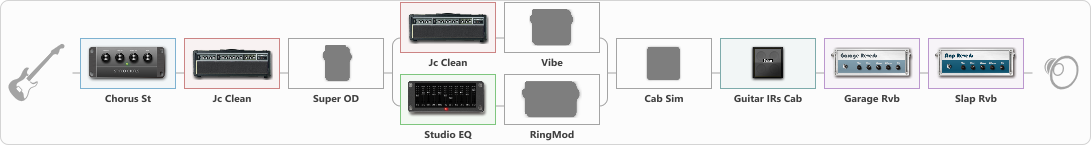# Hendriks met ks preset

Discussion in 'ToneLib-GFX presets' started by Noel Hendriks, Feb 12, 2021.

1. Hendriks met ks preset

Preset name: Clean

Effects chain:Effect: "Chorus St" (Modulation / Sfx), active - "yes"
{
"Speed" = 1.9
"Depth" = 31
"Center" = 4.4
"Mix" = 40
}

Effect: "Jc Clean" (Amp simulators), active - "yes"
{
"Gain" = 27
"Bass" = 96
"Middle" = 31
"Treble" = 88
"Presence" = 39
"Master" = 72
"Level (dB)" = 2
}

Effect: "Super OD" (Overdrive / Distortion), active - "no"
{
"Drive" = 0
"Tone" = 100
"Level" = 50
}

Effect: "Splitter" (Dynamics / Filter), active - "yes"
{
"A-Bypass" = Off
"A-Pan" = 0
"A-Level" = 55
"B-Bypass" = Off
"B-Pan" = 0
"B-Level" = 55

'A' branch:
{

Effect: "Jc Clean" (Amp simulators), active - "yes"
{
"Gain" = 21
"Bass" = 100
"Middle" = 14
"Treble" = 51
"Presence" = 62
"Master" = 64
"Level (dB)" = 6
}

Effect: "Vibe" (Modulation / Sfx), active - "no"
{
"Speed" = 1.9
"Depth" = 17
"Mix" = 70
}
}
'B' branch:
{

Effect: "Studio EQ" (Dynamics / Filter), active - "yes"
{
"31 Hz" = 6
"62 Hz" = -5
"125 Hz" = 9
"250 Hz" = 10
"500 Hz" = 3
"1 kHz" = 7
"2 kHz" = 8
"4 kHz" = 6
"8 kHz" = 9
"16 kHz" = 7
"Level (dB)" = 0
}

Effect: "RingMod" (Modulation / Sfx), active - "no"
{
"Direct" = 11
"Effect" = 17
"Cutoff" = 8.9
"Osc" = 0.0
}
}
}

Effect: "Cab Sim" (Cabinets), active - "no"
{
"Model" = 4x12" 1960 T75
"Level (dB)" = -4
}

Effect: "Guitar IRs Cab" (Cabinets), active - "yes"
{
"Model" = Fender Deluxe Rev (1x12")
"Mic Position" = Middle
"Mic Distance" = Near
"Low Cut (Hz)" = 60
"Hi Cut (kHz)" = 15.5
"Mix" = 100
"Level (dB)" = 2
}

Effect: "Garage Rvb" (Reverberation), active - "yes"
{
"Time" = 4.4
"PreDelay" = 39
"LoDamp" = 3
"HiDamp" = 54
"Mix" = 43
}

Effect: "Slap Rvb" (Reverberation), active - "yes"
{
"Time" = 6.3
"PreDelay" = 30
"LoDamp" = 37
"HiDamp" = 65
"Mix" = 46
}

Note: You will need to download and install the ToneLib-GFX software to use the preset.

File size:
1.1 KB
Views:
2,728